# Motion and Recombination of Electrons and Holes

\begin{align*} \newcommand{\dif}{\mathop{}\!\mathrm{d}}\\ \newcommand{\p}{\partial}\\ \newcommand{\bd}{\boldsymbol}\\ \end{align*}

# 2.1 Thermal Motion

$\frac{\int f(E)D(E)(E-E_c) \dif E}{\int f(E)D(E)\dif E} = \frac{3}{2} kT$

$v_\text{th} = \sqrt{\frac{3kT}{m}}\\ m 取 m_n 或 m_p$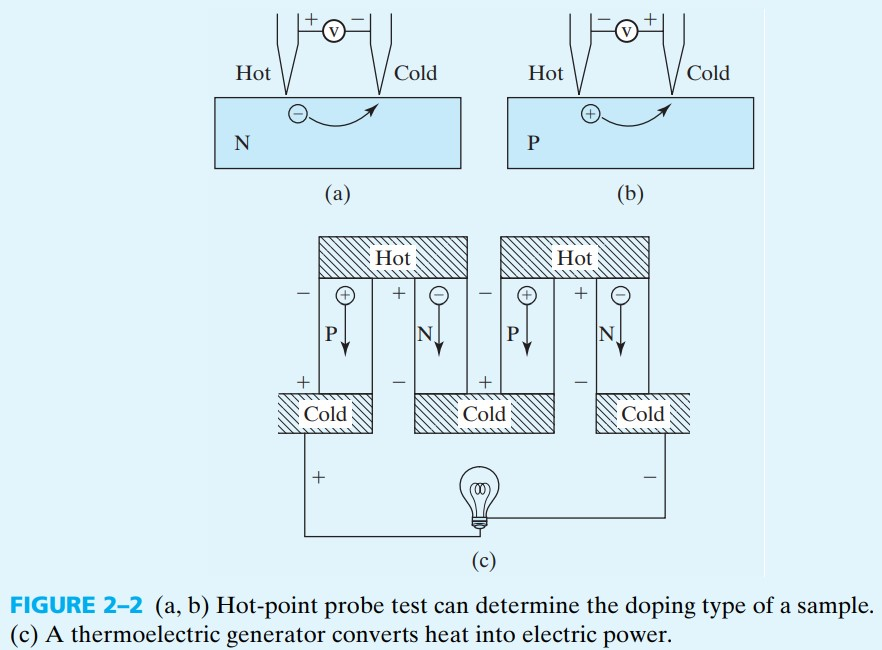# 2.2 Drift

Drift （漂移运动）指载流子在电场下的运动

## 2.2.1 Electron and Hole Mobilities

$m_p v = q\mathscr{E} \tau_\text{mp}$

$\begin{cases} v_p =\mu_p \mathscr{E}\\ \mu_p = \dfrac{q \tau_\text{mp}}{m_p} \end{cases} \tag{2.2.3a}$ $\begin{cases} v_n = - \mu_n \mathscr{E}\\ \mu_n = \dfrac{q \tau_\text{mn}}{m_n} \end{cases} \tag{2.2.3b}$

Si Ge GaAs InAs
$\mu_n$ 1400 3900 8500 30,000
$\mu_p$ 470 1900 400 500

## 2.2.2 Mechanisms of Carrier Scattering

1. phonon scattering 声子散射

Phonons are the particle representation of the vibration of the atoms in the crystal

2. ionized impurity scattering 电离杂质散射

$\frac{1}{\tau} = \frac{1}{\tau_\text{phonon}} + \frac{1}{\tau_\text{impurity}}\\ \frac{1}{\mu} = \frac{1}{\mu_\text{phonon}} + \frac{1}{\mu_\text{impurity}}$

$\tau_\text{phonon}$ 和 $\tau_\text{impurity}$ 与温度的关系如下：

$\mu_\text{phonon} \propto \tau_\text{phonon} \propto T^{-3/2}\\ \mu_\text{impurity} \propto \tau_\text{impurity} \propto \frac{T^{3/2}}{N_a+N_d}$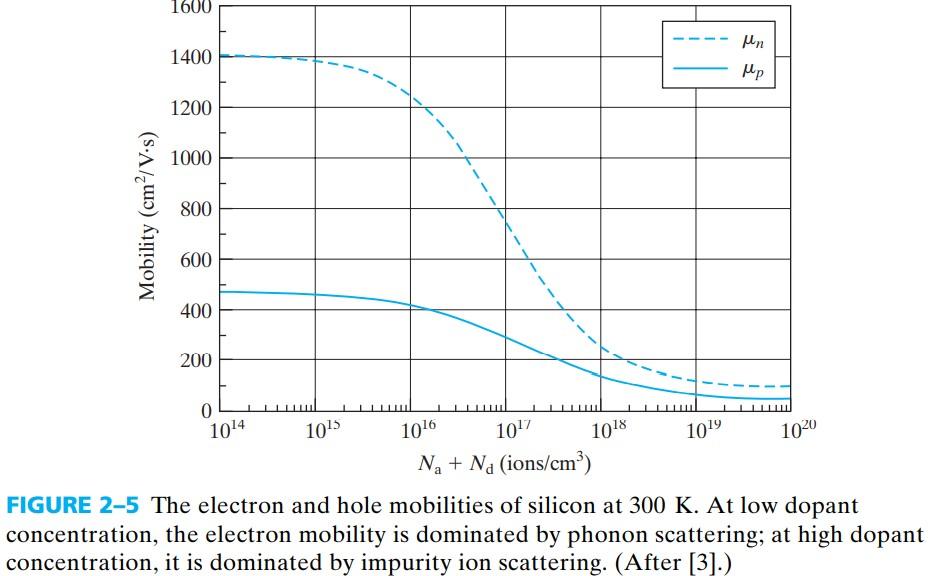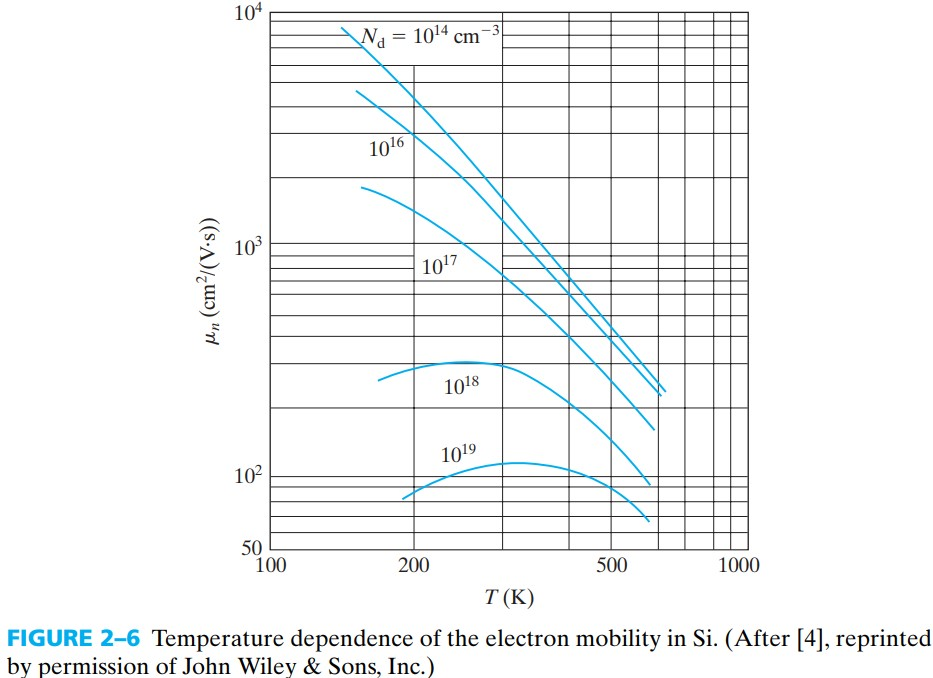## 2.2.3 Drift Current and Conductivity

$J_{p,\text{drift}} = qpv = qp \mu_p \mathscr{E} \tag{2.2.11}$ $J_{n,\text{drift}} = qnv = qn \mu_n \mathscr{E} \tag{2.2.12}$

$J_\text{drift} = J_{p,\text{drift}} + J_{n,\text{drift}}= (qp \mu_p + qn \mu_n )\mathscr{E}$

# 2.3 Diffusion Current

Diffusion is the result of particles undergoing thermal motion，一般来说热运动总是有使各处浓度一致的倾向，当浓度不一致时，热运动就会导致高浓度向低浓度扩散。因此，扩散电流与浓度梯度有关：

$J_{n,\text{diffusion}} = q D_n \frac{\dif n}{\dif x} \tag{2.3.2}\\$ $J_{p,\text{diffusion}} =- q D_p \frac{\dif p}{\dif x} \tag{2.3.3}$

$D_n,D_p$ 称为 diffusion constant. 比较令人困惑的就是上两式中，空穴反而需要加负号？！这是因为梯度本身有一个负号，所以空穴反而要加负号才是正电荷电流。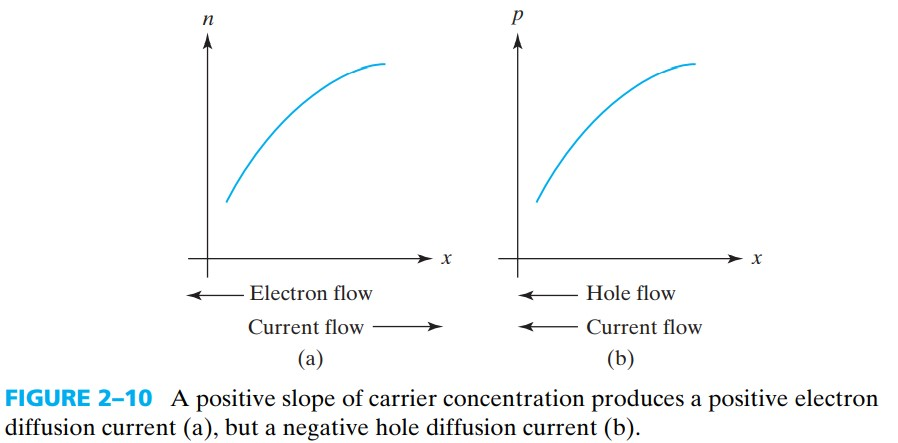$J_n = J_{n,\text{drift}} + J_{n,\text{diffusion}} = qn \mu_n \mathscr{E} + q D_n \frac{\dif n}{\dif x}\\ J_p = J_{p,\text{drift}} + J_{p,\text{diffusion}} = qp \mu_p \mathscr{E} - q D_p \frac{\dif p}{\dif x}\\ J=J_n+J_p$

# 2.4 Relation between the Energy Diagram and V,E

The electrons roll downhill like stones in the energy band diagram and the holes float up like bubbles.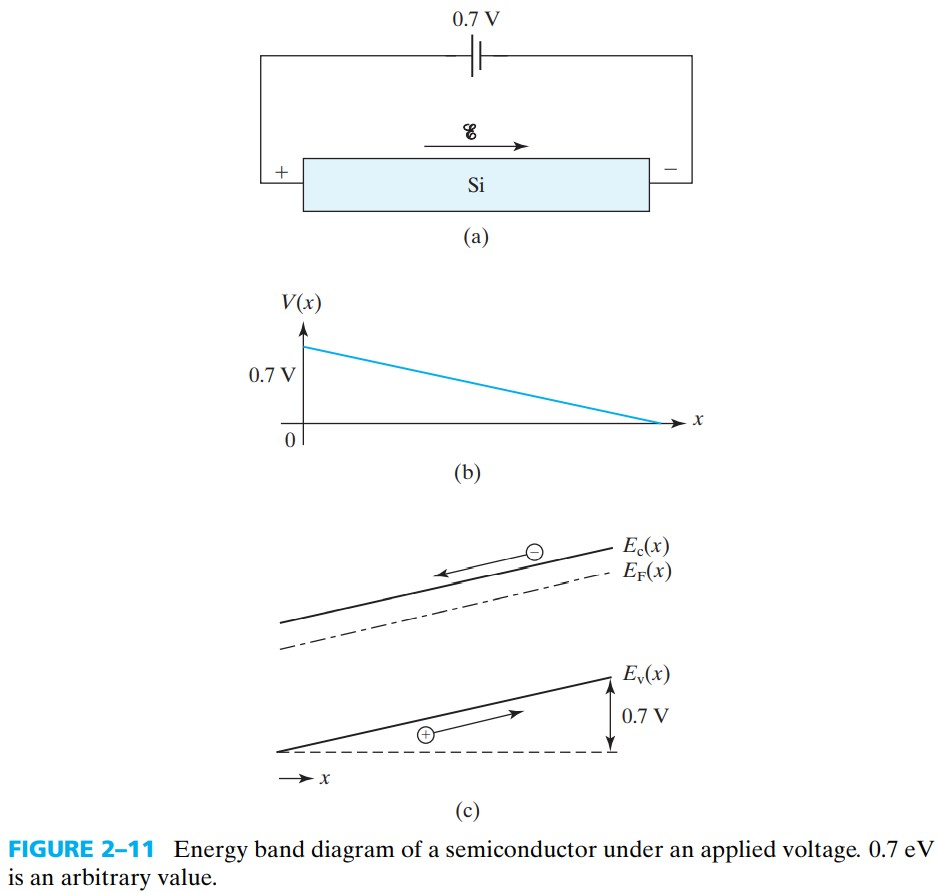$E_c(x)=\text{constant} - qV(x)$

constant 的值由参考电位来决定，而由于 $E_c$ 的单位是 eV，所以 $V(x)$ 要乘上 $q$

$\mathscr{E}(x)=-\frac{\dif V}{\dif x}=\frac{1}{q} \frac{\dif E_c}{\dif x}=\frac{1}{q} \frac{\dif E_v}{\dif x}$

# 2.5 Einstein Relationship between D and μ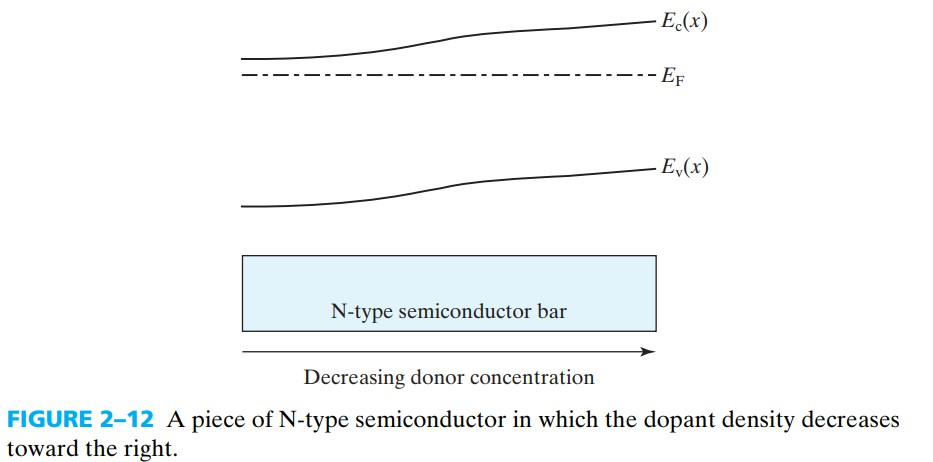$J_n = qn\mu_n \mathscr{E} +q D_n \frac{\dif n}{\dif x} = 0$

$0 = q n \mu_n \mathscr{E} - qn\frac{qD_n}{kT} \mathscr{E}\\ \Rightarrow D_n = \frac{kT}{q} \mu_n \tag{2.5.6a}$

$D_p = \frac{kT}{q}\mu_p \tag{2.5.6b}$

# 2.6 Electron-Hole Recombination

$n'\equiv p' \tag{2.6.2}\\ n \equiv n_0 + n'\\ p \equiv p_0 + p'$

$\frac{\dif n'}{\dif t} = -\frac{n'}{\tau}=-\frac{p'}{\tau}$

recombination rate 结合律 为 $\dfrac{n’}{\tau}=\dfrac{p’}{\tau}$（单位：$/(\text{cm}^3\cdot s)$ ）

1. 直接复合 direct recombination, or radiative recombination：导带电子与价带空穴直接结合
• direct gap semiconductors: efficient. used for light emission.
• indirect gap semiconductors: inefficient. the electrons and holes at the edges of the band gap do not have the same wave vectors
2. 间接复合：导带电子与价带电子在 recombination centers 处复合
3. 俄歇复合？？？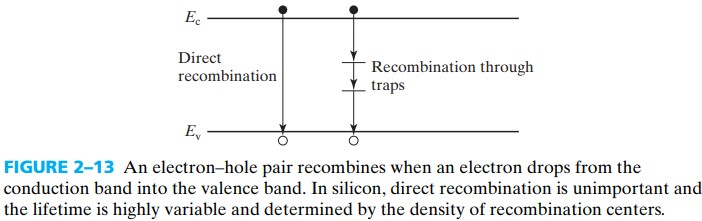# 2.7 Thermal Generation

The reverse process of recombination is called thermal generation 热激发，显然，过剩载流子产生的速度等于 rate of generation minus rate of recombination

# 2.8 Quasi-Equilibrium and Quasi-Fermin Levels

$n = N_c e^{-(E_c-E_{F_n})/kT} \tag{2.8.1}\\$ $p = N_v e^{-(E_{F_p}-E_v)/kT} \tag{2.8.2}$